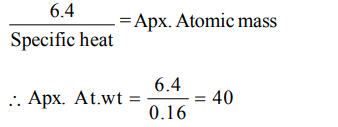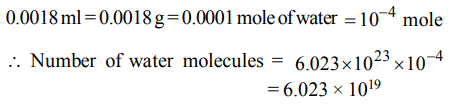## Some Basic Concepts of Chemistry Questions and Answers Part-2

1.7.5 grams of a gas occupy 5.6 litres of volume at STP. The gas is
a) $N_{2}O$
b) NO
c) CO
d) $CO_{2}$

Explanation:2. 1 amu is equal to
a) $\frac{1}{14} of O-16$
b) $\frac{1}{12} of C-12$
c) $1g of H_{2}$
d) $1.66 × 10^{-23}kg$

Explanation: 1 amu = $\frac{1}{12}$ of the mass of C-12

3. Which of the following contains maximum number of atoms?
a) $6.023 × 10^{21}molecules ofCO_{2}$
b) 0.44 g of $CO_{2}$
c) 22.4 L of $CO_{2}$ at STP
d) None of these

Explanation: 22.4 L of CO2 at STP = 1 mole = 6.023 × 1023 molecules. Hence number of atoms 3 × 6.023 × 1023

4. Number of g of oxygen in 32.2 g $Na_{2}SO_{4}.10H_{2}O$    is
a) 20.8
b) 2.24
c) 22.4
d) 2.08

Explanation: M. Wt of Na2SO4 .10 H2O is 322 g which contains 224 g oxygen.
32.2 g will contain 22.4 g oxygen

5. The specific heat of a metal is 0.16, its approximate atomic weight would be
a) 32
b) 16
c) 64
d) 40

Explanation:6. The weight of a molecule of the compound $C_{60}H_{122}$   is
a) $1.09 × 10^{-21}g$
b) $1.4 × 10^{-21}g$
c) $5.025 × 10^{23}g$
d) $16.023 × 10^{23}g$

Explanation:7. The number of water molecules present in a drop of water (volume 0.0018 ml) density = $18 ml^{-1}$ at room temperature is
a)$1.084 × 10^{18}$
b) $6.023 × 10^{19}$
c) $4.84 × 10^{17}$
d) $6.023 × 10^{23}$

Explanation:8. The percentage of Se in peroxidase anhydrous enzyme is 0.5% by weight (atomic weight = 78.4). Then minimum molecular weight of peroxidase anhydrous enzyme is
a) $1.568 × 10^{3}$
b) $1.568 × 10^{4}$
c) 15.68
d) $3.136 × 10^{4}$

Explanation:9. Equivalent weight of crystalline oxalic acid is
a) 90
b) 53
c) 63
d) 45